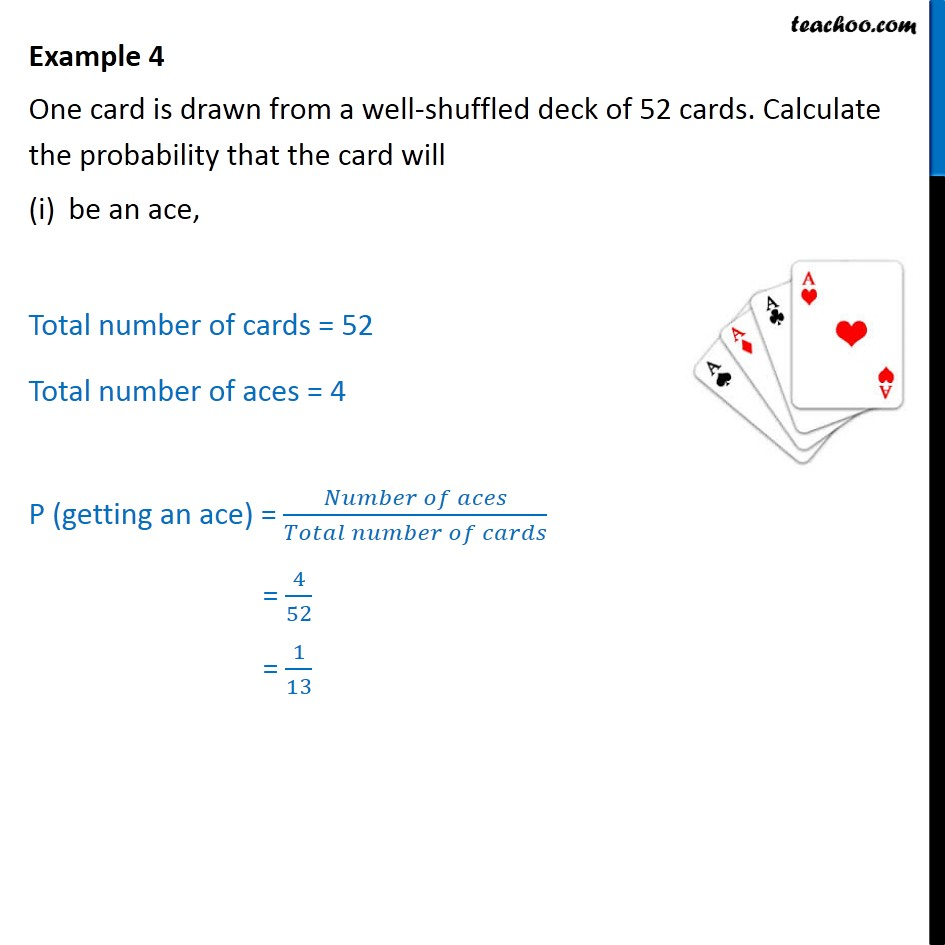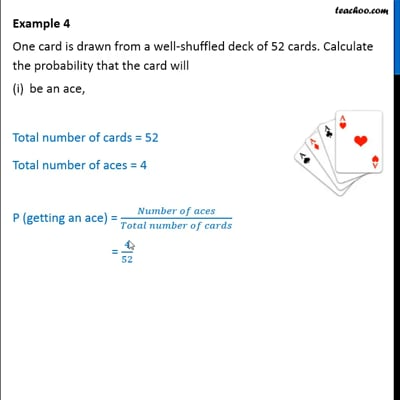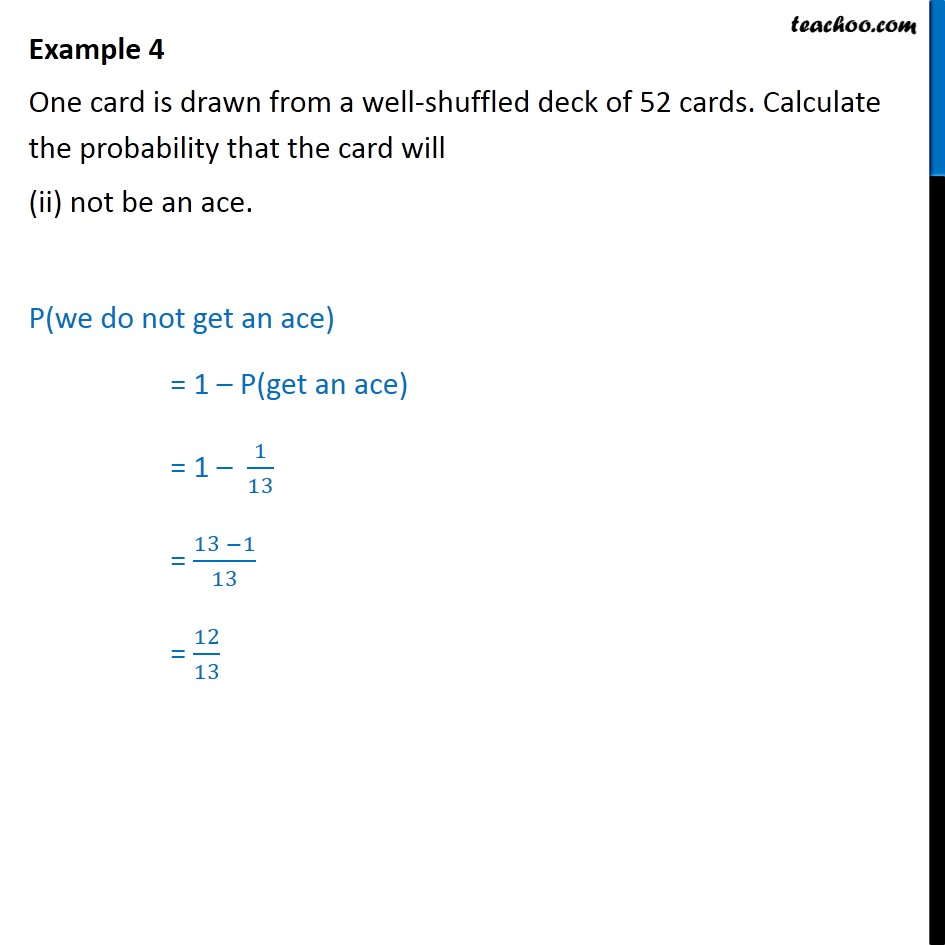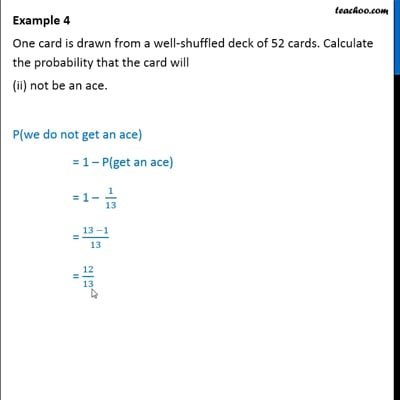Examples

Chapter 15 Class 10 Probability (Term 1)
Serial order wiseThis video is only available for Teachoo black usersThis video is only available for Teachoo black users

### Transcript

Example 4 One card is drawn from a well-shuffled deck of 52 cards. Calculate the probability that the card will be an ace, Total number of cards = 52 Total number of aces = 4 P (getting an ace) = ( )/( ) = 4/52 = 1/13 Example 4 One card is drawn from a well-shuffled deck of 52 cards. Calculate the probability that the card will (ii) not be an ace. P(we do not get an ace) = 1 P(get an ace) = 1 1/13 = (13 1)/13 = 12/13